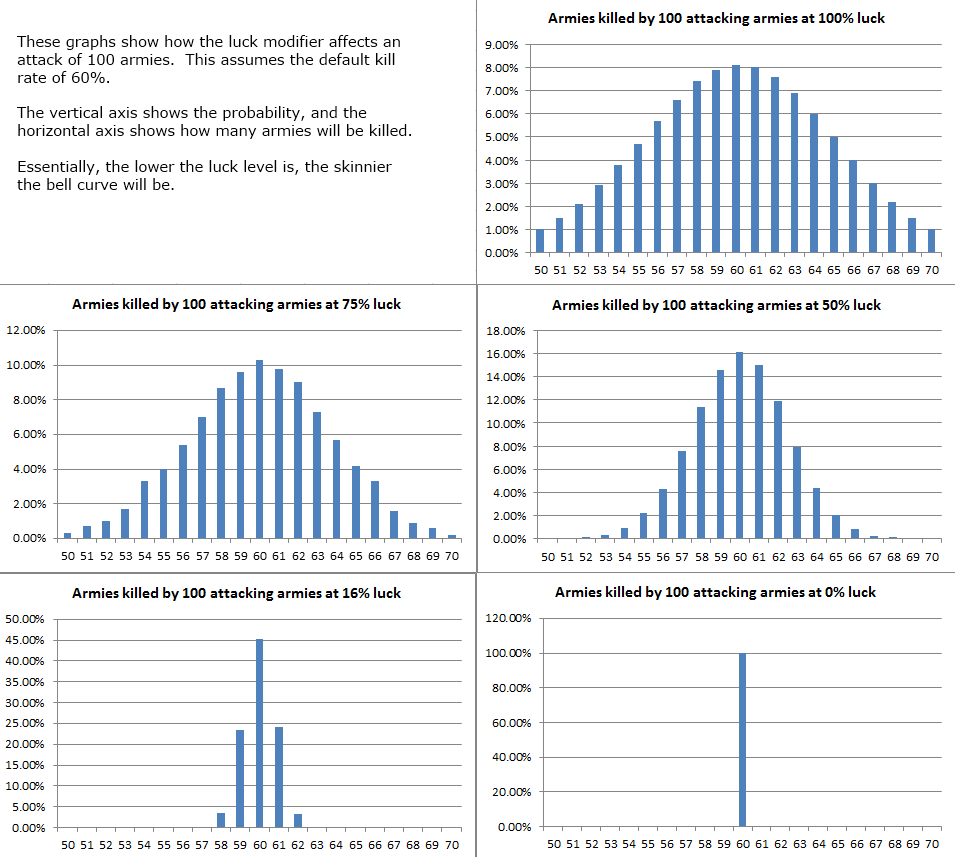# Luck Modifier

(Redirected from Luck modifier)

When a game is created, players have the option to control how much luck will affect the game. They can pick an amount from 0% to 100%. The default is 0%.

Note: Selecting Weighted Random instead of Straight Round also affects the number of armies needed to take a territory.

## Details

To understand the details of how the luck percentage affects combat, it is assumed you first understand the combat basics.

When an attack happens, Warzone calculates the "expected" number of armies that should get killed, assuming an average roll. The attack is also randomized as normal and these two numbers are interpolated based on the luck percentage.

## Example

If a player attacks with 100 armies, it is expected that it will kill 60 of the defending armies, but let's assume the random number generator determined that only 54 would get killed.

In a game with a luck percentage of 100%, Warzone will simply use the 54. However, in a game where the luck percentage had been reduced to 50%, Warzone would take the average of these numbers and would use 57 instead. If the luck percentage were 0%, Warzone would simply ignore the random and use the 60 every single time.

## Analysis## Remainders

In the event that the calculations do not come out evenly, the rounding mode will be used to determine how the result is rounded.